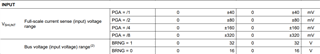If you have a related question, please click the "Ask a related question" button in the top right corner. The newly created question will be automatically linked to this question.

# INA219: Programming the INA219 Calibration Register

Part Number: INA219

I am just getting into the workings of the INA219, but am having trouble understanding the function of the Calibration Register and how it works in general.

1. if I understand correctly, I can select a full scale range of 16 or 32 V. This would then be resolved with 12 bits to determine the bus voltage. This would then be resolved with 12 bits to determine the bus voltage. The lowest voltage resolution would therefore be approximately 7.8 mV or 3.9 mV. However, if the 16 or 32 V are the only reference potential, the shunt voltage must also be referred to this reference. A resolution of 3.9 mV seems extremely high to me, because with an assumed current of 2 A and a shunt resistor of 0.1 Ohm, the voltage drop at the shunt resistor would be only 200 mV. So I would have only 50 steps at my disposal? Am I making a mistake here?
2. the current in the current register is internally calculated by the formula: (shunt_voltage * calibration)/4096. But as I understand it, Calibration is a rather arbitrary value, because it depends on the estimated value of the maximum current. But how can a calibration value depend on an estimated value? For example, if I choose an assumed maximum current of 1 A and a shunt resistor of 0.1 Ohm, I get a calibration value of 13421... but if I assume a maximum current of 2 A, it is 6710... How can an assumed value have such a large influence on my measurement result. Again, the question: Where is my thinking error.

I would be very grateful for any assistance!

Best regards,

Werner Boecker

• Hi Werner,

1. Full scale range of 16 or 32 V is regarding Bus voltage only. The shunt voltage is sperate, with 4 programmable input ranges, 40, 80, 160 and 320mV. To measure current of 2 A and a shunt resistor of 0.1 Ohm (voltage drop 200 mV), I would choose 320mV range. The shunt resolution is 10uV.2. Calibration is rather arbitrary to some extent, you're correct, because it depends on the estimated value of the maximum current. But once you selected the shunt resistor and current LSB (or max current), it is fixed. As long as it is not over-range, the value is used by the device to calculate current. You don’t have to use it however. You could simply divide the shunt voltage by the shunt resistor to get the current value. That way you don't need to read the current register. You can refer to this video for more details on using digital power monitors, including programming the calibration register. The examples are for other devices, but the concept carries over.

Regards, Guang

• Hi Guang,

thanks a lot for your explanation. It is getting much clearer now, but you mentioned a video, but I can´t find any link to it?

Best regards,

Werner

• Hi Werner,